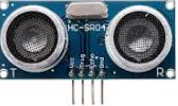# B4R LibraryrHCSR04 - Ultrasonic Distance Sensor

rHCSR04 is an open source B4R library for reading the distance from the Ultrasonic Distance Sensor HC-SR04.rHCSR04
rrHCSR04.zip archive contains the B4R library and B4R sample projects.

Install
From the zip archive, copy the content of the library folder, to the B4R additional libraries folder keeping the folder structure.
Folder structure:
<path to b4r additional libraries folder>\rHCSR04
rHCSR04.h and rHCSR04.cpp
Documentation files
<path to b4r additional libraries folder>
rHCSR04.xml

Hardware
Tested the library with an Arduino UNO and a HC-SR04.

Wiring
HC-SR04 = Arduino UNO
B4X:
``````VCC = 5V (RED)
Trig = 13 (GPIO13) (YELLOW)
Echo = 12 (GPIO12) (GREEN)
GND = GND (BLACK)``````

Functions

Initialize Sensor Object

B4X:
``Initialize(TriggerPin As Byte, EchoPin As Byte)``
Init the object. The sensor reads from 2cm to 400cm (accuracy 0.3cm).
TriggerPin - Pin number of the trigger
EchoPin - Pin number of the echo received
Returns
None
Example
B4X:
``````Private DistanceSensor As HCSR04
Private TriggerPinNr As Byte = 13
Private EchoPinNr As Byte  = 12
DistanceSensor.Initialize(TriggerPinNr, EchoPinNr)``````

Initialize Sensor Object with a Callback Event
B4X:
``Initialize2(DistanceChangedSub As SubVoidDouble, DistanceChangedBy As Double, TriggerPin As Byte, EchoPin As Byte)``
Init the object with callback event. The sensor reads from 2 to 400cm (accuracy 0.3cm).
DistanceChangedSub - Sub to handle distance changes.
DistanceChangedBy - Min Distance changed absolute value to set callback value in distancechangedsub.
TriggerPin - Pin number of the trigger.
EchoPin - Pin number of the echo.
Returns
None
Example
B4X:
``````Private DistanceSensor As HCSR04
Private TriggerPinNr As Byte = 13
Private EchoPinNr As Byte  = 12
DistanceSensor.Initialize2("DistanceChanged", 1.0, TriggerPinNr, EchoPinNr)

' Handle distance changes >= DISTANCECHANGEDBY value
Private Sub DistanceChanged(Distance As Double)
Log("DistanceChanged Data: ", Distance)
End Sub``````

Get the Distance in cm
B4X:
``Distance``
Returns
Distance in cm or -1 if error.
Example
B4X:
``````Private DistanceSensor As HCSR04
' Get the distance (in cm) from the sensor
Dim Distance As Double = DistanceSensor.Distance;
' Log the distance without handling innaccurate values
Log("Distance (cm): ",Distance)``````

Get the Distance in Inch
B4X:
``DistanceInch``
Returns
Distance in Inches or -1 if error.
Example
B4X:
``````Private DistanceSensor As HCSR04
' Get the distance (in Inches) from the sensor
Dim Distance As Double = DistanceSensor.Distance;
' Log the distance without handling innaccurate values
Log("Distance (Inch): ",Distance)``````

B4R Basic Example
B4X:
``````Sub Process_Globals
Public SerialLine As Serial
Public DistanceSensor As HCSR04
Public TriggerPinNr As Byte = 13
Public EchoPinNr As Byte  = 12
Public DistanceCm, DistanceInch As Double
End Sub

Private Sub AppStart
SerialLine.Initialize(115200)
Log("AppStart")
DistanceSensor.Initialize(TriggerPinNr, EchoPinNr)
'Get the distance
DistanceCm = DistanceSensor.Distance
DistanceInch = DistanceSensor.DistanceInch
'Log the distance without handling innaccurate values
Log("Distance: ",DistanceCm, " cm, ", DistanceInch, " Inch")
End Sub``````

B4R Timer Example
B4X:
``````Sub Process_Globals
Public SerialLine As Serial
Public DistanceSensor As HCSR04
Public TriggerPinNr As Byte = 13
Public EchoPinNr As Byte  = 12
Public DistanceTimer As Timer
Public DistanceTimerInterval As Long = 2000
Public DistanceCm, DistanceInch As Double
End Sub

Private Sub AppStart
SerialLine.Initialize(115200)
DistanceSensor.Initialize(TriggerPinNr, EchoPinNr)
DistanceTimer.Initialize("DistanceTimer_Tick", DistanceTimerInterval)
DistanceTimer.Enabled = True
End Sub

Private Sub DistanceTimer_Tick
DistanceCm = DistanceSensor.Distance
DistanceInch = DistanceSensor.DistanceInch
Log("Distance: ",DistanceCm, " cm, ", DistanceInch, " Inch")
End Sub``````

B4R Event Example
B4X:
``````Sub Process_Globals
Public Serial1 As Serial
Public DistanceSensor As HCSR04
Private TriggerPinNr As Byte = 13
Private EchoPinNr As Byte  = 12
Private DISTANCECHANGEDBY As Double = 2.0
End Sub

Private Sub AppStart
Serial1.Initialize(115200)
DistanceSensor
DistanceSensor.Initialize2("DistanceChanged", DISTANCECHANGEDBY, TriggerPinNr, EchoPinNr)
End Sub

' Handle distance changes >= DISTANCECHANGEDBY value
Private Sub DistanceChanged(Distance As Double)
Log("DistanceChanged Data: ", Distance)
End Sub``````

Licence

ToDo
See file TODO.md.

Changelog
v1.30 (20210728) - Event DistanceChanged, DistanceInch.
See file CHANGELOG.md.

#### Attachments

• rHCSR04-130.zip
21.6 KB · Views: 173
Last edited: